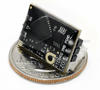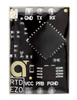EZO™ RTD Temperature Circuit - Atlas Scientific# EZO™ RTD Temperature Circuit - Atlas Scientific

Available: In Stock

### EZO™ RTD Temperature Circuit - Atlas Scientific

The Atlas Scientific EZO™ RTD Temperature circuit is a small footprint computer system that is specifically designed to be used in robotic applications where the embedded systems engineer requires accurate and precise measurements of temperature through a generic PT-100/PT-1000 temperature probe.

RTD = Resistance Temperature Detector

PT = Platinum

PT-100 = 100 Ω at 0˚C

PT-1000 = 1k Ω at 0˚C

### EZO™ RTD Temperature Circuit - Atlas Scientific

The Atlas Scientific EZO™ RTD Temperature circuit is a small footprint computer system that is specifically designed to be used in robotic applications where the embedded systems engineer requires accurate and precise measurements of temperature through a generic PT-100/PT-1000 temperature probe.

RTD = Resistance Temperature Detector

PT = Platinum

PT-100 = 100 Ω at 0˚C

PT-1000 = 1k Ω at 0˚C